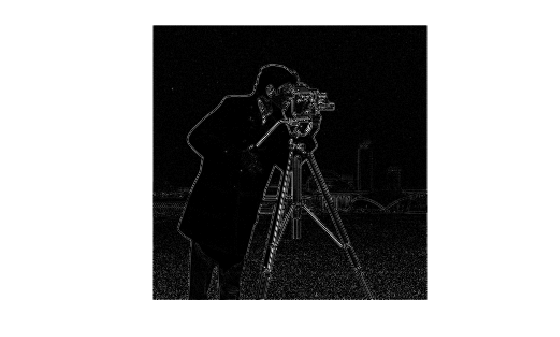# imabsdiff

Absolute difference of two images

## Syntax

``Z = imabsdiff(X,Y)``

## Description

example

````Z = imabsdiff(X,Y)` subtracts each element in array `Y` from the corresponding element in array `X` and returns the absolute difference in the corresponding element of the output array `Z`.```

## Examples

collapse all

`I = imread('cameraman.tif');`

Filter the image.

`J = uint8(filter2(fspecial('gaussian'), I));`

Calculate the absolute difference of the two images.

`K = imabsdiff(I,J);`

Display the absolute difference image.

```figure imshow(K,[])```## Input Arguments

collapse all

Input image, specified as a numeric array of any dimension.

Example: `x = imread('cameraman.tif');`

Data Types: `single` | `double` | `int8` | `int16` | `int32` | `uint8` | `uint16` | `uint32` | `logical`

Input image, specified as a numeric array. `Y` must be the same size and class as `X`.

Data Types: `single` | `double` | `int8` | `int16` | `int32` | `uint8` | `uint16` | `uint32` | `logical`

## Output Arguments

collapse all

Difference image, returned as a numeric array. `Z` has the same class and size as `X` and `Y`. If `X` and `Y` are integer arrays, then `imabsdiff` truncates elements in the output that exceed the range of the integer type.

## Tips

• If `X` is of class `double`, then use the expression `abs(X-Y)` instead of this function.

• If `X` is of class `logical`, then use the expression `XOR(X,Y)` instead of this function.

• When `X` and `Y` are of class `uint8`, `int16`, or `single`, then `imabsdiff` can use hardware optimization to run faster.

## Version History

Introduced before R2006a